# Pre-K and Kindergarten Math Worksheets

#### Where to Start When Learning Math for the First Time

A child starts learning mathematics the moment he/ she starts exploring the world. From identifying shapes to counting them and finding patterns, everything builds on what they know already. A child aged 1-2 can start by understanding the numbers and what they mean. And when asked how old they are, ask them to use fingers to show how many years they are. The next step is to recite numbers, and it's okay if the child skips some of them. Understand the words that measure or compare things (faster, under, behind) Explore the measurements by filling and emptying containers. Match basic shapes like a circle to circle and triangle to triangle. Start seeing patterns in daily routines and things like floor tiles.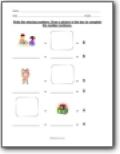###### Math Sentences

Write the missing numbers. Draw a picture in the box to complete the number sentence.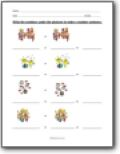###### Number Sentences

Write the numbers under the pictures to make a number sentence.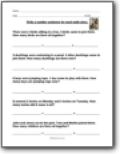###### Word Problem Breakdown

Write a number sentence for each math story.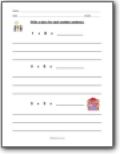###### Math Stories

Write a story for each number sentence.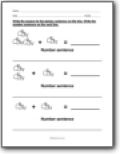###### Picture Sentences

Write the answer to the picture sentence on the line. Write the number sentence on the next line.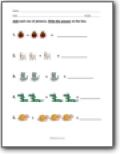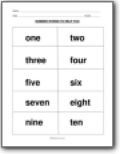###### Number Words 1-10

This is a nice block of the words in bold print for you.###### Introducing Numbers

Look at the number. Write the number word on the line.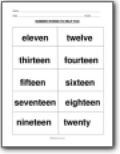###### Number Words 11-20

The words for all those numbers.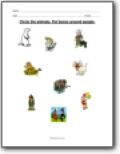###### Circle Things With...

Circle the things that are round. Put boxes around things you eat.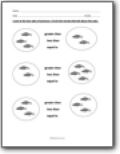###### Comparing Sets of Objects

Look at the two sets of pictures. Circle the words that tell about the sets.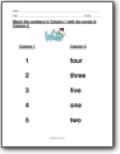###### Number Names

Match the numbers in Column 1 with the words in Column 2.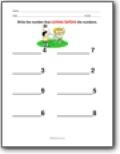###### Missing Numbers

Read the numbers. Write the missing numbers on the lines. Write the number that comes between the numbers.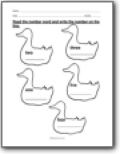###### Words to Numbers

Read the number word and write the number on the line.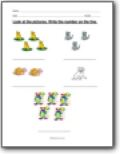###### Pictures to Numbers

Look at the pictures. Write the number on the line.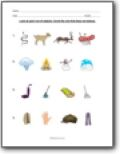###### Which Doesn't Belong?

Look at each row of objects. Circle the one that does not belong.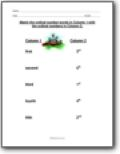###### Ordinal Numbers

Match the ordinal number words in Column 1 with the ordinal numbers in Column 2. Look at the pictures. Using the words under each picture, complete the sentences.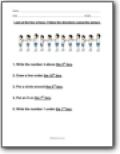###### Follow Directions with Ordinal Numbers

Look at the line of boys. Follow the directions using this picture.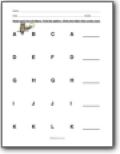###### Patterns in Letters

Read each line of letters. Find the pattern. Write the next set in the pattern.